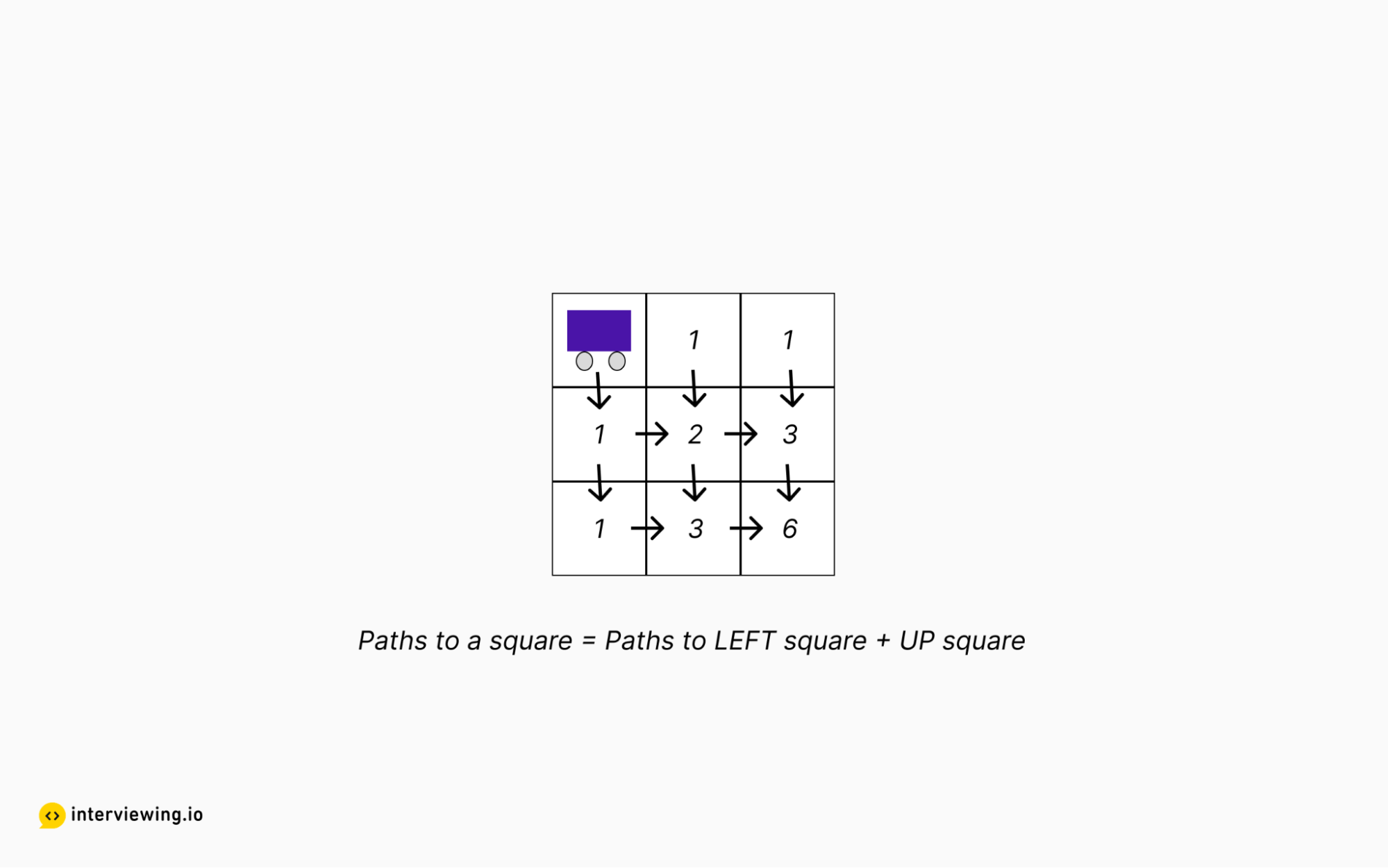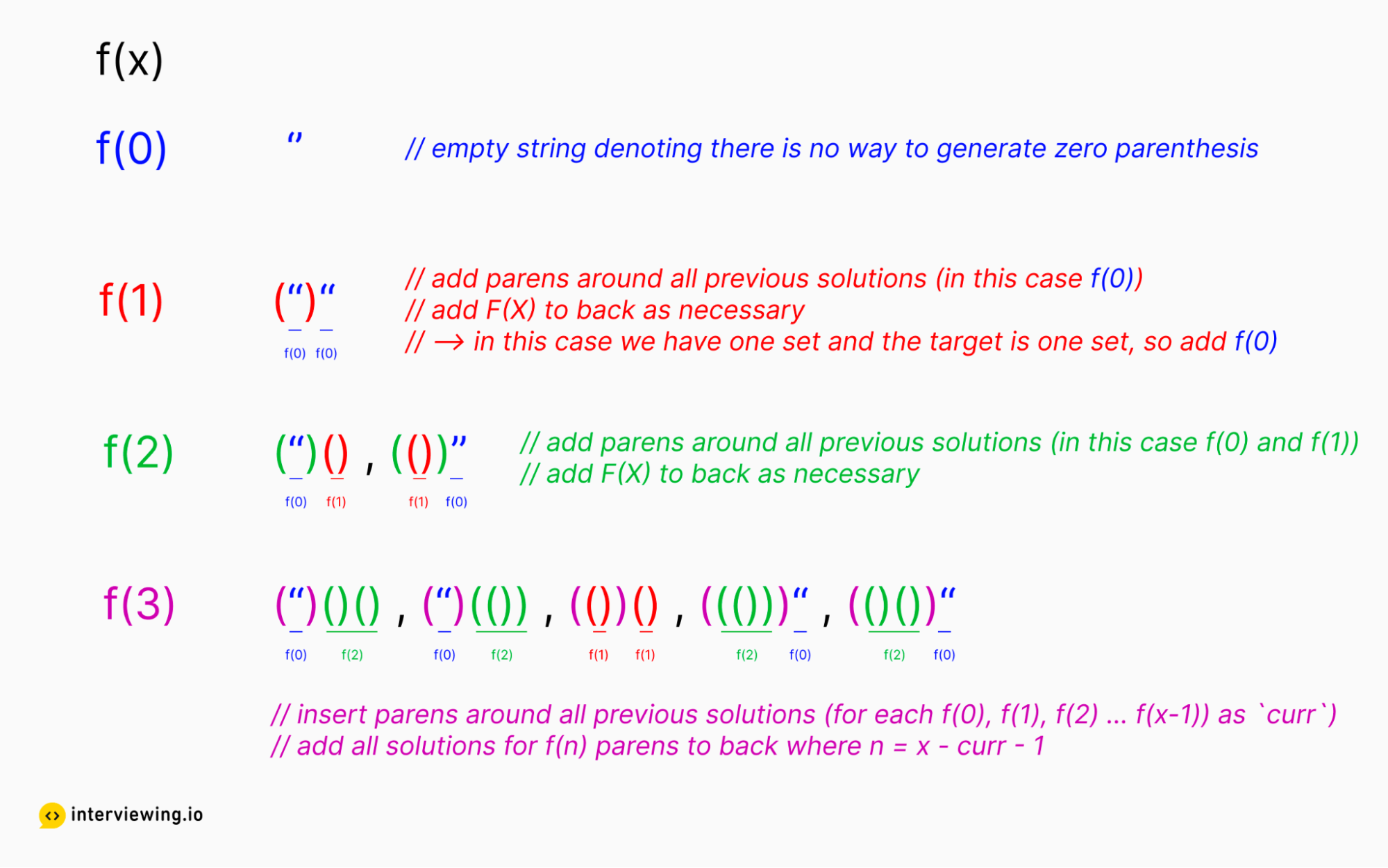MEDIUM
DATA STRUCTURES AND ALGORITHMS

# A Guide to the Generate Parentheses Problem (with Solutions in Python, Java & JavaScript)

Written ByTom WagnerKenny Polyak

## The Generate Parentheses Problem: An Overview

The Generate Parenthesis problem involves generating all valid combinations of parentheses for a given number of pairs of parenthesis. This problem can be solved using both recursive and iterative approaches, and is a great introduction to backtracking style recursion.

## An Example of the Generate Parentheses Problem

Given `n` pairs of parentheses, write a function to generate all combinations of well-formed parentheses.

Input: n = 3 Output: ["((()))","(()())","(())()","()(())","()()()"]

Input: n = 2 Output: [“()()”, “(())”]

Constraints

• `1 <= n <= 10,000`

## How to Solve the Generate Parentheses Problem: 2 Approaches

Below we walk through two different ways to solve the generate parentheses problem: using recursion and dynamic programming.

### Approach 1: Recursion

We are tasked with generating all `combinations` of random parenthesis, and the word `combinations` should serve as a clue that recursion could be useful. Let’s say we are solving this problem by hand for `n = 2`. Logically, we start with one forward paren `(`, and from here we have two options: add another forward paren, or add a backward paren.

Note that we will not always be able to add a forward or backward paren. If we are generating all combinations for `n = 2` and we already have two forward parens, then we shouldn’t try to add more forward parens. Likewise, if we only have one forward paren in the solution thus far but we already have one backward paren, we can’t add any more backwards parentheses as that would make our solution invalid.

Putting it all together we have a recursive solution where we build up and tear down possible solutions, adding parens as we go. We modify a list containing the forward and backward parens instead of concatenating strings because string concatenation is a linear time complexity operation, while appending to and popping from a list can be done in constant time.

#### Generate Parentheses Python, JavaScript and Java Solutions - Recursion

``````class Solution:
def generateParenthesis(self, n: int) -> List[str]:
current_string = []

def recurse(forward_parens_needed: int, backward_parens_needed: int) -> None:
nonlocal current_string

if forward_parens_needed == 0 and backward_parens_needed == 0:

if forward_parens_needed > 0:
current_string.append('(')
recurse(forward_parens_needed - 1, backward_parens_needed)

if backward_parens_needed > 0 and forward_parens_needed < backward_parens_needed:
current_string.append(')')
recurse(forward_parens_needed, backward_parens_needed - 1)

if len(current_string) > 0:
current_string.pop()

recurse(n, n)
``````

#### Time/Space Complexity

• Time complexity: `O(2n)`
• Space complexity: `O(n)`, as we never have more than `n` * 2 parentheses in the `valid_answers` array.

### Approach 2: Dynamic Programming

When solving an algorithm problem and looking for a more efficient solution, dynamic programming can often be a great tool to improve the time complexity of a solution, though spotting the pattern and implementing the code can be tricky.

To spot a place where dynamic programming could be helpful, we look for situations where solving a subproblem (or a few subproblems) can help solve the main problem at hand. For example, consider a simpler problem in which we are trying to determine the number of ways a robot can traverse a grid from the top left corner to the bottom right corner while only going down and to the right.

To get to a given square, the robot can either move to the right from the square on the left or move down from the square above. Thus, the number of ways the robot can get to square `[x][y]` = routes to `[x - 1][y]` + routes to `[x][y - 1]`.Turning back to generate parentheses, the dynamic programming pattern is more difficult to spot. At a high level, we solve for `generateParenthesis(0)`, `generateParenthesis(1)` ... `generateParentheses(X - 1)` and then combine those solutions to solve for `generateParentheses(X)`.For each value of `X`, we insert a new pair of parentheses around all possible solutions to `generateParentheses(0)` ... `generateParentheses(X - 1)`, and then combining each of those solutions with however many parentheses we still need in order to generate `X` parentheses total. By the time we get to `n` we have solved this problem for every number between 0 and `n`, with the answers stored in a zero-indexed array, so we simply return the `nth` index in the array.

This particular problem is a fairly difficult dynamic programming problem, so if you are new to algorithms and/or dynamic programming I would suggest focussing on simpler problems such as the robot in grid problem mentioned above first, then coming back to this problem once solidifying your understanding of the basics.

#### Generate Parentheses Python, JavaScript and Java Solutions - Dynamic Programming

``````class Solution:
def generateParenthesis(self, n: int) -> List[str]:
dp = [[] for i in range(n + 1)]
dp.append('')

# iterate over all f(0) ... f(n) parenthesis pair targets
for i in range(n + 1):
# iterate over all solutions generated thus far --> f(0) ... f(i)
for j in range(i):
# iterate over all solutions for f(j)
for x in dp[j]:
# iterate over all solutions for f(n - j)
for y in dp[i - j - 1]:
# insert solution for f(j) between parens
# --> and add all solutions for f(n - j) to back
to_be_added.append('(' + x + ')' + y)

return dp[n]``````

#### Time/Space Complexity

• Time complexity: `O(n * Cat(n))`, where `Cat` represents the time complexity of Catalan numbers (out of scope for this article and likely for an interview).
• Space complexity: `O(n * Cat(n))`.

## Practice the Generate Parentheses Problem With Our AI Interviewer

Start AI Interview